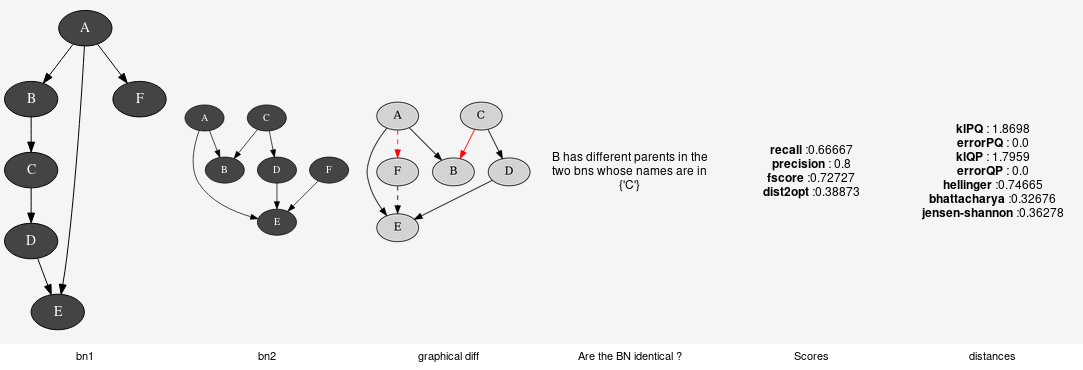Comparison of Bayesian networks¶To compare Bayesian network, one can compare the structure of the BNs (see pyAgrum.lib.bn_vs_vb.GraphicalBNComparator). However BNs can also be compared as probability distributions.

class pyAgrum.ExactBNdistance(*args)

Class representing exacte computation of divergence and distance between BNs

ExactBNdistance(P,Q) -> ExactBNdistance
Parameters:
• P (pyAgrum.BayesNet) a Bayesian network
• Q (pyAgrum.BayesNet) another Bayesian network to compare with the first one
ExactBNdistance(ebnd) -> ExactBNdistance
Parameters:
• ebnd (pyAgrum.ExactBNdistance) the exact BNdistance to copy
Raises: gum.OperationNotAllowed – If the 2BNs have not the same domain size of compatible node sets
compute(ExactBNdistance self)
Returns: a dictionnary containing the different values after the computation. dict
class pyAgrum.GibbsBNdistance(*args)

Class representing a Gibbs-Approximated computation of divergence and distance between BNs

GibbsBNdistance(P,Q) -> GibbsBNdistance
Parameters:
• P (pyAgrum.BayesNet) – a Bayesian network
• Q (pyAgrum.BayesNet) – another Bayesian network to compare with the first one
GibbsBNdistance(gbnd) -> GibbsBNdistance
Parameters:
• gbnd (pyAgrum.GibbsBNdistance) – the Gibbs BNdistance to copy
Raises: gum.OperationNotAllowed – If the 2BNs have not the same domain size of compatible node sets
burnIn(GibbsBNdistance self)
Returns: size of burn in on number of iteration int
compute(GibbsBNdistance self)
Returns: a dictionnary containing the different values after the computation. dict
continueApproximationScheme(ApproximationScheme self, double error)

Continue the approximation scheme.

Parameters: error (double) –
currentTime(GibbsBNdistance self)
Returns: get the current running time in second (double) double
disableEpsilon(ApproximationScheme self)

Disable epsilon as a stopping criterion.

disableMaxIter(ApproximationScheme self)

Disable max iterations as a stopping criterion.

disableMaxTime(ApproximationScheme self)

Disable max time as a stopping criterion.

disableMinEpsilonRate(ApproximationScheme self)

Disable a min epsilon rate as a stopping criterion.

enableEpsilon(ApproximationScheme self)

Enable epsilon as a stopping criterion.

enableMaxIter(ApproximationScheme self)

Enable max iterations as a stopping criterion.

enableMaxTime(ApproximationScheme self)

Enable max time as a stopping criterion.

enableMinEpsilonRate(ApproximationScheme self)

Enable a min epsilon rate as a stopping criterion.

epsilon(GibbsBNdistance self)
Returns: the value of epsilon double
history(GibbsBNdistance self)
Returns: the scheme history tuple gum.OperationNotAllowed – If the scheme did not performed or if verbosity is set to false
initApproximationScheme(ApproximationScheme self)

Initiate the approximation scheme.

isDrawnAtRandom(GibbsBNdistance self)
Returns: True if variables are drawn at random bool
isEnabledEpsilon(ApproximationScheme self)
Returns: True if epsilon is used as a stopping criterion. bool
isEnabledMaxIter(ApproximationScheme self)
Returns: True if max iterations is used as a stopping criterion bool
isEnabledMaxTime(ApproximationScheme self)
Returns: True if max time is used as a stopping criterion bool
isEnabledMinEpsilonRate(ApproximationScheme self)
Returns: True if epsilon rate is used as a stopping criterion bool
maxIter(GibbsBNdistance self)
Returns: the criterion on number of iterations int
maxTime(GibbsBNdistance self)
Returns: the timeout(in seconds) double
messageApproximationScheme(GibbsBNdistance self)
Returns: the approximation scheme message str
minEpsilonRate(GibbsBNdistance self)
Returns: the value of the minimal epsilon rate double
nbrDrawnVar(GibbsBNdistance self)
Returns: the number of variable drawn at each iteration int
nbrIterations(GibbsBNdistance self)
Returns: the number of iterations int
periodSize(GibbsBNdistance self)
Returns: the number of samples between 2 stopping int gum.OutOfLowerBound – If p<1
remainingBurnIn(ApproximationScheme self)
Returns: the number of remaining burn in int
setBurnIn(GibbsBNdistance self, int b)
Parameters: b (int) – size of burn in on number of iteration
setDrawnAtRandom(GibbsBNdistance self, bool _atRandom)
Parameters: _atRandom (bool) – indicates if variables should be drawn at random
setEpsilon(GibbsBNdistance self, double eps)
Parameters: eps (double) – the epsilon we want to use gum.OutOfLowerBound – If eps<0
setMaxIter(GibbsBNdistance self, int max)
Parameters: max (int) – the maximum number of iteration gum.OutOfLowerBound – If max <= 1
setMaxTime(GibbsBNdistance self, double timeout)
Parameters: tiemout (double) – stopping criterion on timeout (in seconds) gum.OutOfLowerBound – If timeout<=0.0
setMinEpsilonRate(GibbsBNdistance self, double rate)
Parameters: rate (double) – the minimal epsilon rate
setNbrDrawnVar(GibbsBNdistance self, int _nbr)
Parameters: _nbr (int) – the number of variables to be drawn at each iteration
setPeriodSize(GibbsBNdistance self, int p)
Parameters: p (int) – number of samples between 2 stopping gum.OutOfLowerBound – If p<1
setVerbosity(GibbsBNdistance self, bool v)
Parameters: v (bool) – verbosity
startOfPeriod(ApproximationScheme self)
Returns: True if it is a start of a period bool
stateApproximationScheme(ApproximationScheme self)
Returns: the state of the approximation scheme int
stopApproximationScheme(ApproximationScheme self)

Stop the approximation scheme.

updateApproximationScheme(ApproximationScheme self, unsigned int incr=1)

Update the approximation scheme.

verbosity(GibbsBNdistance self)
Returns: True if the verbosity is enabled bool